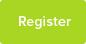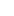# Tutor Hunt Questions

You need to add a salt to a DNA solution. At the moment the DNA is dissolved in water, you need it to be in 0.2 M sodium acetate. The volume of the DNA solution is 1.9 ml and you have a stock 2 M sodium acetate.
How much sodium acetate do you need to add to the DNA solution to bring the final concentration to 0.2 M sodium acetate?

Thank you
4 years ago

Please enter your response to the question below. The student will get a notification as soon your response has been approved by our moderation team.

0.2M is required (0.2 M = 0.0002 mol/ml). c=n/v where n= moles and v =volume. The stock solution has a conc. of 2M( = 0.002 mol/ml). Let x = ml of stock added.

Now n = cv. (n = 0.002x, v = 1.9 + x). Required Conc. = 0.0002 mol/ml

So, 0.0002 = n/v = 0.002x/(1.9 + x) . Solve the equation for x.

Algebraically x = 0.211 ml of stock added.
Using n=cv (where n= amount, c=concentration and v=volume)
Initial concentration of acetate (c1)=2M
Final " " (c2)=0.2M

This means there is a dilution factor of 10.

As no extra amount is added than that used, n is constant. And take the volume added to be x, and the total volume with the DNA 1.9+x in ml.

This means that c1x=c2(1.9+x)=====> 1.8x=0.2(1.9)

Gives answer to be: x = 0.211 ml
19ml
We need to find the volume of salt solution (sodium acetate) for the proportions to be 9 part DNA and 1 part Salt.
(0.2/2 = 1/10, 1-1/10 = 9/10)
1.9ml is equal to 9/10 of the solution, therefore
1/10 = 1.9/9 = 0.211ml (3dp)
The easiest way to answer this type of question is by remembering the following formula:

c1 x v1 = c2 x v2

where c1 = initial (or stock) concentration, c2 = final concentration, v1 = initial volume, and v2 = final volume.

Based on your question c1= 2M, v1 = unknown, c2 = 0.2M, and v2 = (1.9ml + v1)

So if you put all those numbers into the equation above:

2M x v1 = 0.2M x (1.9ml + v1)

rearrange the equation to solve v1:

2M x v1 = (0.2M x 1.9ml) + (0.2M x v1)
2M x v1 = 0.38M/ml + (0.2M x v1)
(2M x v1) - (0.2M x v1) = 0.38M/ml
v1(2M-0.2M) = 0.38M/ml
v1 x 1.8M = 0.38M/ml
v1 = 0.38M/ml / 1.8M
v1 = 0.211ml